4WX Internet Search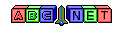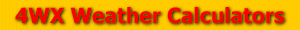# Wind Chill

From the user, we are given an air temperature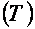and a wind speed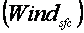In order to calculate the Wind Chill, the temperature must be converted to degrees Fahrenheit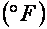.

To find out how to convert the temperature, see the link below:

tempConvert.php

Also, in order to calculate the Wind Chill, the wind speed must be converted to miles per hour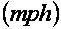.

To find out how to convert the wind speed, see the link below:

Then, the Wind Chill can be calculated using this formula:Because the user might need the Wind Chill in Watts per meters squared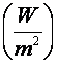, it can calculated using an air temperature in degrees Celsius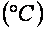and a wind speed in meters per second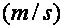:Back to 4WX Weather Calculators index page

4WX Calculators Credit and disclaimer